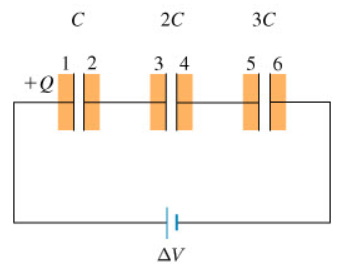# Problem: (a) Find the voltage, V1 across the first capacitor. Express your answer in terms of V.(b) Find the charge Q on the first capacitor. Express your answer in terms of C and V1.(c) Using the value of Q just calculated, find the equivalent capacitance Ceq for this combination of capacitors in series. Express your answer in terms of C.

###### FREE Expert Solution

(a) Capacitance, C is given as;

$\overline{){\mathbf{C}}{\mathbf{=}}\frac{\mathbf{Q}}{\mathbf{V}}}$

Total voltage is given by:

$\overline{){\mathbf{∆}}{\mathbf{V}}{\mathbf{=}}{\mathbf{∆}}{{\mathbf{V}}}_{{\mathbf{1}}}{\mathbf{+}}{\mathbf{∆}}{{\mathbf{V}}}_{{\mathbf{2}}}{\mathbf{+}}{\mathbf{∆}}{{\mathbf{V}}}_{{\mathbf{3}}}}$

90% (107 ratings)###### Problem Details

(a) Find the voltage, V1 across the first capacitor. Express your answer in terms of V.

(b) Find the charge Q on the first capacitor. Express your answer in terms of C and V1.

(c) Using the value of Q just calculated, find the equivalent capacitance Ceq for this combination of capacitors in series. Express your answer in terms of C.Frequently Asked Questions

What scientific concept do you need to know in order to solve this problem?

Our tutors have indicated that to solve this problem you will need to apply the Capacitors & Capacitance concept. You can view video lessons to learn Capacitors & Capacitance. Or if you need more Capacitors & Capacitance practice, you can also practice Capacitors & Capacitance practice problems.

What professor is this problem relevant for?

Based on our data, we think this problem is relevant for Professor Markert's class at TEXAS.